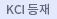#검색
다국어 입력

http://chineseinput.net/에서 pinyin(병음)방식으로 중국어를 변환할 수 있습니다.

변환된 중국어를 복사하여 사용하시면 됩니다.

예시)
• 中文 을 입력하시려면 zhongwen을 입력하시고 space를누르시면됩니다.
• 北京 을 입력하시려면 beijing을 입력하시고 space를 누르시면 됩니다.
닫기

검색결과 좁혀 보기

• 좁혀본 항목 보기순서

• 원문유무
• 음성지원유무
• 원문제공처
• 등재정보
• 학술지명
• 주제분류
• 발행연도
• 작성언어
• 저자

오늘 본 자료

• 오늘 본 자료가 없습니다.
더보기
• 무료
• 기관 내 무료
• 유료
•ASYMPTOTIC STABILITY AND DECAY RATE OF SOLUTIONS FOR A NONLINEAR FOURTH ORDER WAVE EQUATION

This paper is concerned with investigating the global as-ymptotic behavior of the zero solution of the initial-boundary value problem for a nonlinear fourth order wave equation, Moreover an esti-mate of the rate of decay of the solutions is obtained.

•A new characterization of alternating andsymmetric groups

In this paper we prove that the alternating groups An, for n = p, p+1, p+2 and symmetric groups Sn, for n = p, p+1, where p 3 is a prime number, can be uniquely determined by their order components. As one of the important consequence of this characterization we show that the simple groups An, where n = p, p+1, p+2 and p 3 is prime, satisfy in Thompson's conjecture and Shi's conjecture.

•Intuitionistic fuzzy congruences on a lattice

We study the relationship between intuitionistic fuzzy ideals and intuitionistic fuzzy congruences on a distributive lattice. And we prove that the lattice of intuitionistic fuzzy ideals is isomorphic to the lattice of intuitionistic fuzzy congruences on a generalized Boolean algebra.

•Normal fuzzy probability for trigonometric fuzzy number

We calculate the normal fuzzy probability for trigonometric fuzzy numbers defined by trigonometric functions. And we study the normal probability for some operations of two trigonometric fuzzy numbers. Furthermore, we calculate the normal fuzzy probability for some fuzzy numbers generated by operations.

•SOCLE ELEMENTS OF NON-LEVEL ARTINIAN ALGEBRAS

We show that an Artinian O-sequence $h_0,h_1,{\cdots},h_{d-1},h_d\;=\;h_{d-1},h_{d+l}\;>\;h_d$ of codimension 3 is not level when $h_{d-1}\;=\;h_d\;=\;d + i\;and\;h{d+1}\;=\;d+(i+1)\;for\;i\;=\;1,\;2,\;and\;3$, which is a partial answer to the question in . We also introduce an algorithm for finding noncancelable Betti numbers of minimal free resolutions of all possible Artinian O-sequences based on the theorem of Froberg and Laksov in .

•PRODUCT PROPERTIES OF DIGITAL COVERING MAPS

The aim of this paper is to solve the open problem on product properties of digital covering maps raised from . Namely, let us consider the digital images $X_1 {\subset}Z^{n_{0}}$ with $k_0-adjacency$, $Y_1{\subset}Z^{n_{1}}$ with $k_3-adjacency$, $X_2{\subset}Z^{n_{2}}$ with $k_2-adjacency$ and $Y_2{\subset}Z^{n_{3}}$ with $k_3-adjacency$. Then the reasonable $k_4-adjacency$ of the product image $X_1{\times}X_2$ is determined by the $k_0-$ and $k_2-adjacency$ and the suitable k_5-adjacency$is assumed on$Y_1{\times}Y_2$via the$k_1-$and$k_3-adjacency$ such that each of the projection maps is a digitally continuous map, e.g.,$p_1\;:\;X_1{\times}X_2{\rightarrow}X_1$is a digitally ($k_4,\;k_1$)-continuous map and so on. Let us assume$h_1\;:\;X_1{\rightarrow}Y_1$to be a digital$(k_0, k_1)$-covering map and$h_2\;:\;X_2{\rightarrow}Y_2$to be a digital$(k_2,\;k_3)$-covering map. Then we show that the product map$h_1{\times}h_2\;:\;X_1{\times}X_2{\rightarrow}Y_1{\times}Y_2$need not be a digital$(k_4,k_5)\$-covering map.

•FUZZY DIFFERENTIAL EQUATIONS WITH NONLOCAL CONDITION

We shall prove the existence and uniqueness theorem of a solution to the non local fuzzy differential equation using the contraction mapping principle.

•SURVEY OF GIBBS PHENOMENON FROM FOURIER SERIES TO HYBRID SAMPLING SERIES

An understanding of Fourier series and their generalization is important for physics and engineering students, as much for mathematical and physical insight as for applications. Students are usually confused by the so-called Gibbs' phenomenon, an overshoot between a discontinuous function and its approximation by a Fourier series as the number of terms in the series becomes indefinitely large. In this paper we give short story of Gibbs phenomenon in chronological order.

•Survey of Gibbs phenomenon from Fourier series to hybrid sampling series

An understanding of Fourier series and their generalization is important for physics and engineering students, as much for mathematical and physical insight as for applications. Students are usually confused by the so-called Gibbs' phenomenon, an overshoot between a discontinuous function and its approximation by a Fourier series as the number of terms in the series becomes indefinitely large. In this paper we give short story of Gibbs phenomenon in chronological order.

•INTUITIONISTIC FUZZY CONGRUENCES ON A LATTICE

We study the relationship between intuitionistic fuzzy ideals and intuitionistic fuzzy congruences on a distributive lattice. And we prove that the lattice of intuitionistic fuzzy ideals is isomorphic to the lattice of intuitionistic fuzzy congruences on a generalized Boolean algebra.